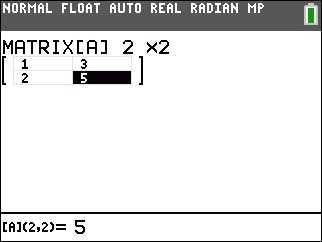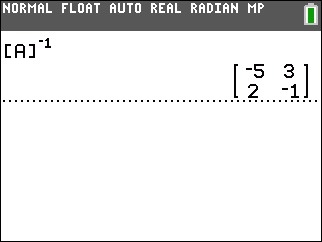# Knowledge Base

## Solution 34852: Calculating the Inverse of a Matrix on the TI-83 Plus and TI-84 Plus Family of Graphing Calculators.

### How do I calculate the inverse of a matrix on the TI-83 Plus and TI-84 Plus family of graphing calculators?

The example below will show how to calculate the inverse of a square matrix.

For Example: Find the inverse of matrix AFirst enter the matrix:
1) Press [2nd] [MATRX] to enter the matrix menu.
2) Press [Left Arrow] to scroll to EDIT.
2) Press  to edit Matrix A
3) Input the dimensions of the matrix  [ENTER]  [ENTER]
4) Input the values for the elements of the matrix  [ENTER]  [ENTER]  [ENTER]  [ENTER]